#### You may also like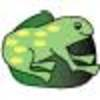### The Lily Pond

Freddie Frog visits as many of the leaves as he can on the way to see Sammy Snail but only visits each lily leaf once. Which is the best way for him to go?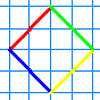### A Cartesian Puzzle

Find the missing coordinates which will form these eight quadrilaterals. These coordinates themselves will then form a shape with rotational and line symmetry.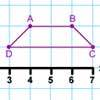### Transformation Tease

What are the coordinates of this shape after it has been transformed in the ways described? Compare these with the original coordinates. What do you notice about the numbers?

# Treasure Island

## Treasure Island

Here is a treasure map.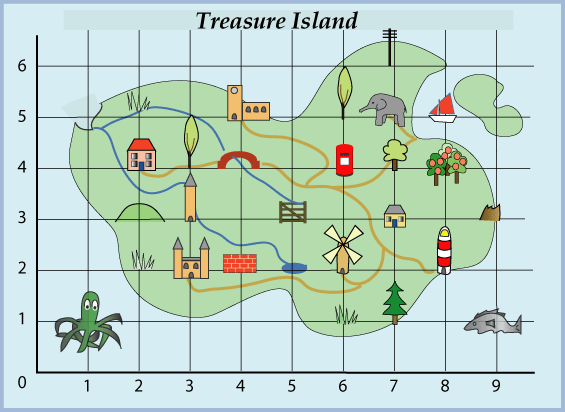What are the co-ordinates of these objects?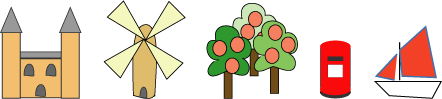Write down what you can see at the co-ordinates given below:

1. ($7, 5$)
2. ($9, 5$)
3. ($5, 3$)
4. ($2, 3$)
5. ($7, 1$)
6. ($3, 3$)
7. ($4, 2$)
8. ($1, 1$)

The words can be used in a special way to find the coordinates of the buried treasure. Can you work out where it is?

### Why do this problem?

This problem is an interesting way of practising using coordinates in the first quadrant. The answer is found in a way which will intrigue able young learners.

### Key questions

What can you find at the point given by these coordinates?
Can you think of another word that might describe this object?
What is the initial letter of this word?
What do these letters spell out?

### Possible extension

Learners could make their own treasure island map on squared giving the coordinates of various objects.

### Possible support

Suggest finding the objects at each of the given coordinates and writing down their names. It is the initial letter of the picture clues which you need to spell out the answer.